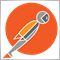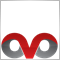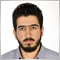# using NotePad++ to edit and compile - page 32466

Carl Schreiber:

Let me add that you can get a function list of your own functions quite easily:

2) add this:  <association userDefinedLangName="mql4" id="mql4_sytax"/>

here:

3) add this in the next section:

(I just copied them from c further below and there is as well one for c++ - may be valid for mql5.)

I f you now click on the Function List button (or in menu => View => Function List) you see a window with the list of all your functions of the actual mql4 or mqh file.

A click on a function and you are there!

Here the example of Statistics.mqh:

You can sort the functions alphabetically :)

Thank you for posting this! I copied the parser for c++ and it now you can view functions by class with mql.

<parser
id         ="mql_syntax" displayName="MQL Class" commentExpr="(?s:/\*.*?\*/)|(?m-s://.*?\$)">
<classRange
mainExpr    ="^[\t\x20]*(class|struct)[\t\x20]+\w+\s*(final)?\s*(:\s*(public|protected|private)\s+\w+\s*)?\{"
openSymbole ="\{"
closeSymbole="\}"
>
<className>
<nameExpr expr="(class|struct)[\t\x20]+\w+" />
<nameExpr expr="[\t\x20]+\w+" />
<nameExpr expr="\w+" />
</className>
<function
mainExpr="^[\t\x20]*((static|const|virtual)\s+)?(\w+(\s+\w+)?(\s+|(\*|\*\*|&amp;)\s+|\s+(\*|\*\*|&amp;)|\s+(\*|\*\*|&amp;)\s+))?(\w+\s*::)?(?!(if|while|for|switch))[\w~]+\s*\([^\)\(]*\)(\s*const\s*)?(\s*(final|override|final\s*override|override\s*final)\s*)?[\n\s]*\{"
>
<functionName>
<funcNameExpr expr="(?!(if|while|for|switch))[\w~]+\s*\(" />
<funcNameExpr expr="(?!(if|while|for|switch))[\w~]+" />
</functionName>
</function>
</classRange>
<function
mainExpr="^[\t\x20]*((static|const|virtual)\s+)?\w+(\s+\w+)?(\s+|(\*|\*\*|&amp;)\s+|\s+(\*|\*\*|&amp;)|\s+(\*|\*\*|&amp;)\s+)(\w+\s*::)?(?!(if|while|for))\w+\s*\([^\)\(]*\)(\s*const\s*)?[\n\s]*\{"
>
<functionName>
<nameExpr expr="(?!(if|while|for))\w+\s*\(" />
<nameExpr expr="(?!(if|while|for))\w+" />
</functionName>
<className>
<nameExpr expr="\w+(?=\s*::)" />
</className>
</function>
</parser>2466

I also forgot to mention, in response to version control and diff view, there are some really cool free tools that I much prefer over the built in MQL5 storage and version control. The first, bitbucket.org allows free private repositories and overall I like it better than github. Next, you're going to need git. The command line is always good, but I've recent fallen in love with the git GUI - SourceTree. Here you can access everything at a quick glance.

You can integrate external diff viewers to work with sourcetree to manage merge conflict and view file diff. I personally like DiffMerge.2926

Emma Schwatson:

Thank you for posting this! I copied the parser for c++ and it now you can view functions by class with mql.

<parser
id         ="mql_syntax" displayName="MQL Class" commentExpr="(?s:/\*.*?\*/)|(?m-s://.*?\$)">
<classRange
mainExpr    ="^[\t\x20]*(class|struct)[\t\x20]+\w+\s*(final)?\s*(:\s*(public|protected|private)\s+\w+\s*)?\{"
openSymbole ="\{"
closeSymbole="\}"
>
<className>
<nameExpr expr="(class|struct)[\t\x20]+\w+" />
<nameExpr expr="[\t\x20]+\w+" />
<nameExpr expr="\w+" />
</className>
<function
mainExpr="^[\t\x20]*((static|const|virtual)\s+)?(\w+(\s+\w+)?(\s+|(\*|\*\*|&amp;)\s+|\s+(\*|\*\*|&amp;)|\s+(\*|\*\*|&amp;)\s+))?(\w+\s*::)?(?!(if|while|for|switch))[\w~]+\s*\([^\)\(]*\)(\s*const\s*)?(\s*(final|override|final\s*override|override\s*final)\s*)?[\n\s]*\{"
>
<functionName>
<funcNameExpr expr="(?!(if|while|for|switch))[\w~]+\s*\(" />
<funcNameExpr expr="(?!(if|while|for|switch))[\w~]+" />
</functionName>
</function>
</classRange>
<function
mainExpr="^[\t\x20]*((static|const|virtual)\s+)?\w+(\s+\w+)?(\s+|(\*|\*\*|&amp;)\s+|\s+(\*|\*\*|&amp;)|\s+(\*|\*\*|&amp;)\s+)(\w+\s*::)?(?!(if|while|for))\w+\s*\([^\)\(]*\)(\s*const\s*)?[\n\s]*\{"
>
<functionName>
<nameExpr expr="(?!(if|while|for))\w+\s*\(" />
<nameExpr expr="(?!(if|while|for))\w+" />
</functionName>
<className>
<nameExpr expr="\w+(?=\s*::)" />
</className>
</function>
</parser>

I am completely uneducated in RE, so it takes me ages to adopt the parser. I am missing a match for constructors, both simple and with the ":" extension. If any one knows how to fix the parser for them, I would appreciate it.

I am also missing the inner classes in the tree, while their functions get assigned to the outer class (like the picture below), however I do not hope the parser can be configured to manage them.2466

Ex Ovo Omnia:

I am completely uneducated in RE, so it takes me ages to adopt the parser. I am missing a match for constructors, both simple and with the ":" extension. If any one knows how to fix the parser for them, I would appreciate it.

I am also missing the inner classes in the tree, while their functions get assigned to the outer class (like the picture below), however I do not hope the parser can be configured to manage them.

Have you tried the vscode editor instead?2926

Emma Schwatson:
Have you tried the vscode editor instead?

No, and I do not plan. Is there some really good reason I should?2466

Ex Ovo Omnia:

No, and I do not plan. Is there some really good reason I should?

Yes. notepad++ is obsolete. I wrote some vscode extensions that not only highlight mql syntax but use the c++ intellisence with MQL so you can do everything you'd expect from an IDE like peek definitions, etc. I'd say give it a try... install vscode, click the extensions tab, search "mql", and install the "MQL Extension pack".31077

https://www.mql5.com/en/forum/21852639

Hello friends

how can copy text to clipboard in mql5?

I tried most of the answers but unfortunately did not get any results.Moderator
21934